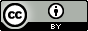Betting odds

Created: 2015-01-27; last major update: 2015-07-20
Status: notes; belief: unlikely

Definition

Betting someone that $$E$$ is true or happens with an odds of $$x$$-to-$$y$$ and a stake of $$\n$$ means that if you lose ($$E$$ is false or does not happen), you pay the $$\n$$ stake. If you win ($$E$$ is true or happens), you keep the stake, and, in addition, win $$\\left(\frac{x}{y}\cdot n\right)$$.

Taking a bet from someone that $$E$$ is true or happens with an odds of $$x$$-to-$$y$$ and a stake of $$\n$$ means that if $$E$$ is false or does not happen, then you collect the $$\n$$ stake. If $$E$$ actually is true or does happen, then you lose and pay $$\\left(\frac{x}{y}\cdot n\right)$$.

The above implies the following for the person betting: if they believe that the the probability of $$E$$ being true or happening is $$p$$, then their expected profit following the bet is

$p\cdot \frac{x}{y}\cdot n - (1-p) \cdot n$

So if $$p = \frac{y}{x+y}$$ precisely, then their expected profit following the bet is

$\frac{y}{x+y}\cdot \frac{x}{y}\cdot n - \frac{x}{x+y}\cdot n = 0$

Similarly, for the person taking the bet, if they believe the probability of $$E$$ being true or happening is $$q$$, then their expected profit following the bet is

$-q \cdot \frac{x}{y} \cdot n + (1-q) \cdot n$

So if precisely $$q = \frac{y}{x+y}$$, the expected profit following the bet is the same as when $$p = \frac{y}{x+y}$$:

$-\frac{y}{x+y}\cdot \frac{x}{y} \cdot n + \frac{x}{x+y}\cdot n = 0$

In other words, if both sides of the bet believe in $$E$$ at the neutral probability derived from the betting odds (i.e. they’re betting at the stated odds), then neither stands to make a profit (in expectation). This is what Eliezer Yudkowsky means when he says:

Taking a bet at 99-1 does not mean I think the probability is 1%. It means I think the probability is enough less than 1% that I stand to make a profit even after adverse selection is taken into account. That is how betting is supposed to work.

When good rationalists are virtuously betting with each other over a disagreement, neither should feel that they are betting at the true odds. If two people both think they are betting at the true odds, they must agree on the odds, so they must agree on the probability, so they must agree on the credibility, so what on Earth are they betting about?

Example 1

We take an example from Noah Smith’s “Bets do not (necessarily) reveal beliefs”.

First, DeLong gives Smith 50-to-1 odds that inflation would go over 5%. Let the stakes for Smith be $$\alpha$$. This means that if inflation goes over 5%, Smith wins, and gets $$50\alpha$$. If inflation stays under 5%, Smith loses, and loses the $$\alpha$$ from the stakes.

Next, Smith gives Chovanec 25-to-1 odds that inflation would stay under 5%. This means that if inflation goes over 5%, Smith loses, and must pay $$25\alpha$$. On the other hand, if inflation stays under 5%, Smith wins, and wins the stakes of Chovanec, namely $$\alpha$$. (Perhaps it’s easier to see this looking from Chovanec’s view: )

This means that, overall, if inflation goes over 5%, then Smith gets: $$50\alpha - 25\alpha = 25\alpha$$, or “25 pizza dinner equivalents”, since $$\alpha$$ was the cost of a pizza dinner. On the other hand, if inflation stays under 5%, then Smith gets: $$-\alpha + \alpha = 0$$, or breaks even.

Example 2

Alex Tabarrok in “A Bet is a Tax on Bullshit” gives the example of Nate Silver betting on the outcome of the presidential election. Tabarrok says:

A properly structured bet is the most credible guarantor of rigorous disinterest. In order to prove his point, Silver is not required to take the Obama side of the bet! At the odds implied by his model (currently between 3 and 4 to 1) Silver should be willing to take either side of a modest bet. Indeed, we could hold a coin toss, heads Silver takes the Obama side, tails he takes Romney.

In fact, the NYTimes should require that Silver, and other pundits, bet their beliefs. Furthermore, to remove any possibility of manipulation, the NYTimes should escrow a portion of Silver’s salary in a blind trust bet. In other words, the NYTimes should bet a portion of Silver’s salary, at the odds implied by Silver’s model, randomly choosing which side of the bet to take, only revealing to Silver the bet and its outcome after the election is over. A blind trust bet creates incentives for Silver to be disinterested in the outcome but very interested in the accuracy of the forecast.

Suppose Silver thinks Obama will win with odd 3-to-1, and suppose he’s willing to stake $$\alpha$$. Now, we can make a tree diagram of all the possibilities:

/\
Obama    /  \   Romney
.5   /    \      .5
/      \
/------/        \------\
/                        \
O wins /\R wins          O wins  /\  R wins

So the expected value is: $\frac{1}{2} \left( \frac{x}{x+y} \cdot \frac{x}{y} \cdot \alpha \\ - \frac{y}{x+y} \cdot \alpha \\ - \frac{x}{x+y} \cdot \alpha \\ + \right)$